# Writing and Forming Algebraic Expressions

Writing and Forming Algebraic Expressions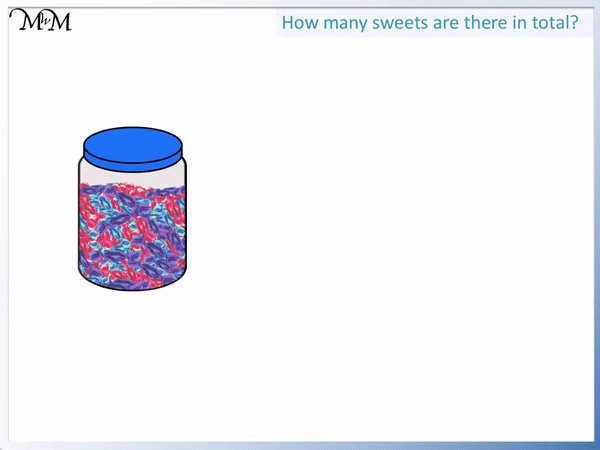• Algebraic expressions can be made up of variables and numbers.
• Variables are the ‘letters’.
• We do not know exactly how many sweets there are in this jar.
• Instead of writing a number, we say that there are ‘n’ sweets in the jar.
• We can use any letter, such as x, y or z but we have chosen ‘n’ in this case.
• As well as the jar, we have 2 more sweets.
• We have n sweets in the jar and 2 more.
• We write the total number of sweets as ‘n + 2’.

We write algebraic expressions when a certain value is unknown.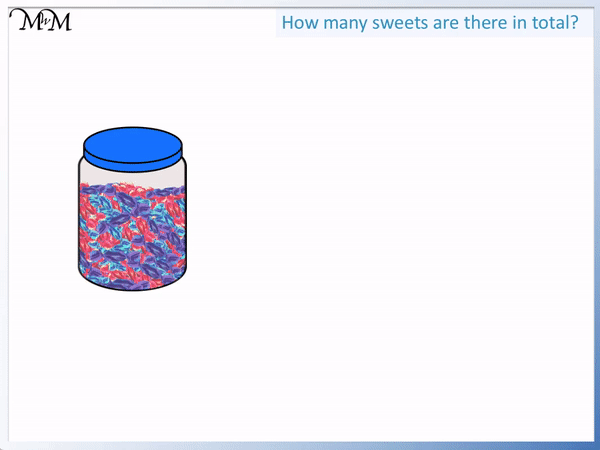• We do not know how many sweets are in the jar.
• We say that there are n sweets in the jar.
• We take one sweet out.
• We had n sweets and we subtracted 1.
• There are now ‘n – 1’ sweets in the jar.# Writing and Forming Algebraic Expressions

## What are Algebraic Expressions?

Algebraic expressions can contain numbers, variables and operations but they must not contain an equals sign or inequality signs.

The value of each algebraic expression depends on the value of the variables inside it. The variables are the algebraic letters. They are called variables because they can vary in value.

We can use algebraic expressions when the value of something is unknown.

For example, we do not know how many sweets are in this jar.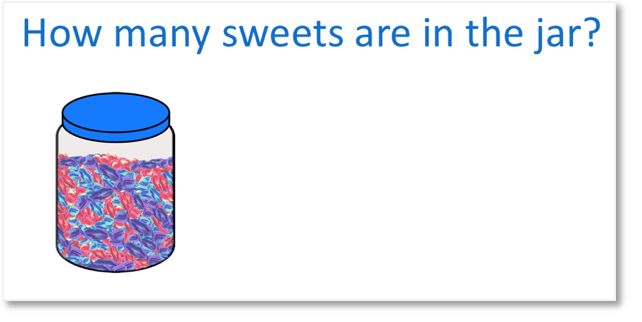We will write a letter to represent how many sweets are inside the jar.

When writing algebraic expressions we can use any letter of the alphabet and we can even use symbols instead.

In this example, we have chosen the lowercase letter n. n is often used in algebraic expressions to represent an unknown number.There are n sweets in the jar.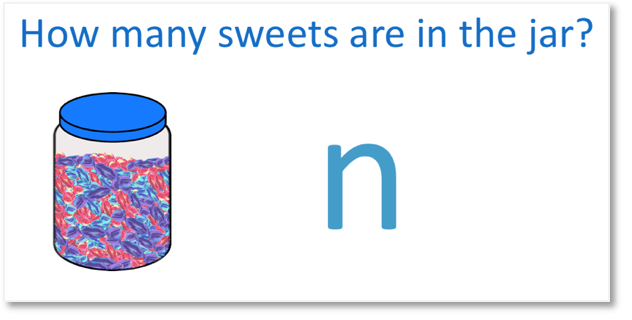Algebraic expressions can also contain numbers as well as variables.

Here we have the same jar of sweets but we are given 2 more sweets.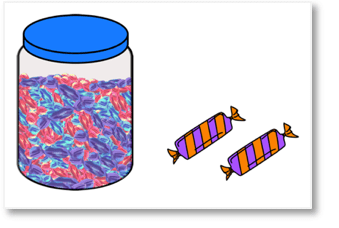We have the original n sweets in the jar plus 2 more. We can translate the words into an algebraic expression.

We now have n + 2 sweets in total.n + 2 is an example of an algebraic expression.

We can see that there are no equals signs in an algebraic expression.

When teaching writing algebraic expressions, it is important to explain that there is no numerical answer to be found. The expression is a mixture of both variables and numbers.The variable is the letter n.

When we find out the number of sweets in the jar then we can put a value on the number of sweets in total.

If there are 100 sweets in the jar, then n = 100 and therefore n + 2 = 100 + 2, which is 102.

Alternatively, if there are 30 sweets in the jar, then n = 30 and therefore n + 2 = 30 + 2, which is 32.

We can only get a numerical answer if we know the value of the the variables (letters) in the expression.

## Forming Algebraic Expressions

Here is a jar of sweets plus 4 more.We do not know how many sweets are in the jar, so we say that there are ‘n’ sweets in the jar.

We have 4 more than the number of sweets in the jar.

When writing algebraic expressions, the words ‘more than’ means to add.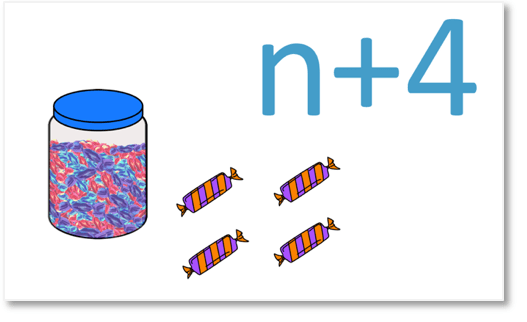We have the n sweets in the jar and 4 more. We can write the algebraic expression as n + 4.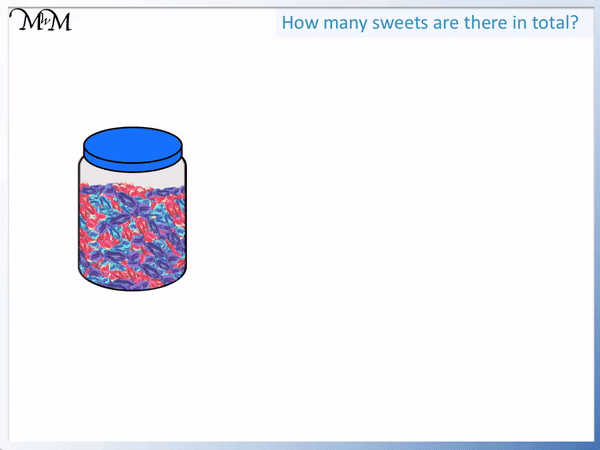In this example we start with the same jar of sweets but this time we take one out.We started with n sweets but subtracted 1.

We translate the words into the algebraic expression of n – 1.We have n – 1 sweets.Here is another example involving subtraction.

This time we start with the jar of n sweets and take out 5 sweets.The algebraic expression for this problem is ‘n – 5’ sweets.In this next example we have two identical jars of sweets. We do not know how many sweets are in these jars.There are the same number of sweets in each jar. Because we don’t know how many sweets are in the jars, we will say that we have n sweets in each jar.We have 2 lots of n, which is 2 × n.

When writing algebraic expressions, we don’t write multiplication signs. Instead of 2 × n, we write 2n.

We simply have 2n sweets.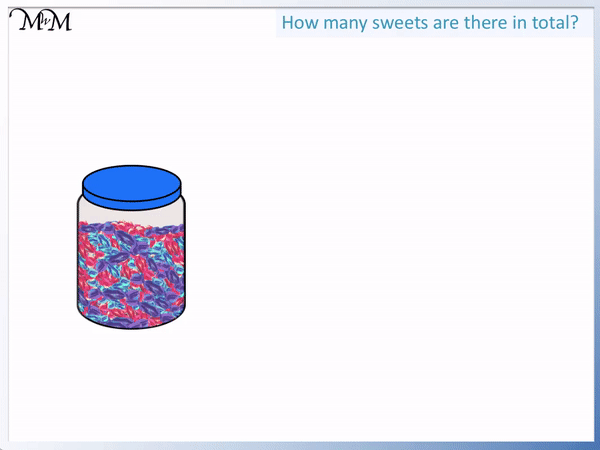In the next example of writing algebraic expressions with multiplication, we have 5 jars of sweets.There are n sweets in each jar and so we have 5 lots of n.

When translating these words into an algebraic expression, we replace the words ‘lots of’ with a multiplication.

We have 5 × n.

In algebra, we don’t write multiplication signs and so, we simply have 5n sweets.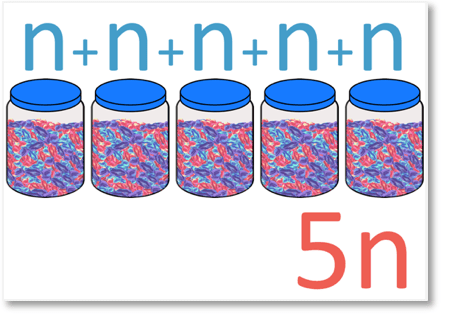The algebraic expression is that there are 5n sweets in total.## The Difference Between an Expression and an Equation

The difference between an expression and an equation is simply that an equation contains an equals sign and an expression does not.

An equation can be solved, whereas an expression cannot. An expression can be evaluated if we know the values of the variables inside it.

An example of an expression is ‘3n’.

There can be numbers and variables (letters) in an expression but no equals sign. We can see in the case of ‘3n’ that there is no equals sign here.

This ‘3n’ example could mean that we have 3 jars of sweets, where each jar has ‘n’ sweets inside.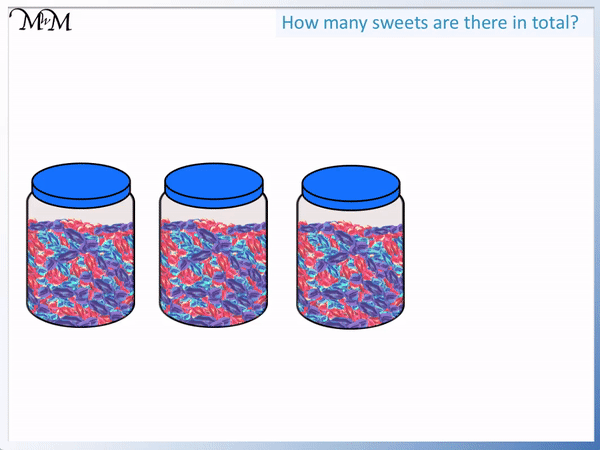An equation contains an equals sign.

For example, ‘3n = 30’ is an example of an equation.

We have an equals sign and the equation can be solved.

We can divide both 3n and 30 by three to see that n = 10.

The equation 3n = 30 is like saying, “We have 3 jars of sweets and in total this makes 30 sweets. How many sweets were in each jar?”

n is the number of sweets in each jar. If there are 3 jars, there must have been 10 sweets in each jar if there are 30 sweets in total. This is because 3 × 10 = 30.

We do not solve expressions.

The expression 3n is simply telling us that we have 3 lots of n. We do not know how many in total so we do not need to work anything out.

When teaching the writing of expressions it is important to point this out because some people expect to find a numerical ‘answer’ to the expression. They often expect to find a number, like we did in solving the equation above to get n = 10.

If the question is simply asking you to write an algebraic expression for the 3 jars of sweets, then ‘3n’ is the answer. There is no way to work anything further out.

However, if we are later told that there are 20 sweets in each jar, then we can evaluate 3n because we would then know that n = 20.

If n = 20, then 3n = 60.

If n = 100, then 3n = 300.

We leave the algebraic expression as 3n, so that we find the value of n at a later stage, we can substitute it in place to work out the total.Now try our lesson on Writing Algebraic Terms where we learn how to write algebraic terms.error: Content is protected !!